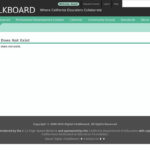# Tag Archives: equations and inequalities#### Linear Functions

Oktober 13th, 2017

In this tutorial, „Linear functions of the form f(x) = a x + b and the properties of their graphs are explored interactively using an applet.“ The applet allows students to manipulate variables to discover the changes in intercepts and slope of the graphed line. There are six questions for students to answer, exploring the […]#### Solving Linear Inequalities: Introduction and Formatting

Oktober 13th, 2017

From Elizabeth Stapel at Purplemath, this module helps students understand how to solve linear inequalities with an introduction to the topic using both inequality notation and a graphic representation to express the inequality.